# MSBSHSE Solutions For Class 8 Maths Part 1 Chapter 7- Variation

MSBSHSE Solutions For Class 8 Maths Part 1 Chapter 7 – Variation is provided here to help students prepare for their exams at ease. Students who face difficulty in understanding the concepts during class hours can quickly refer to MSBSHSE Solutions. The facilitators at BYJU’S have formulated the solutions based on the students’ grasping abilities. This chapter mainly deals with direct variation, inverse variation, Time, Work and Speed concepts. Students can solve exercise wise problems to increase their confidence level before appearing for the board exam. To boost interest among students in this chapter, Maharashtra State Board Class 8 Textbooks Part 1 pdf links are given here for easy access. Students can refer and download the PDF of Maharashtra Board Solutions for Class 8 Maths Chapter 7 Variation, which is readily available for download from the links provided.

## Download the PDF of Maharashtra Board Solutions For Class 8 Maths Part 1 Chapter 7- Variation.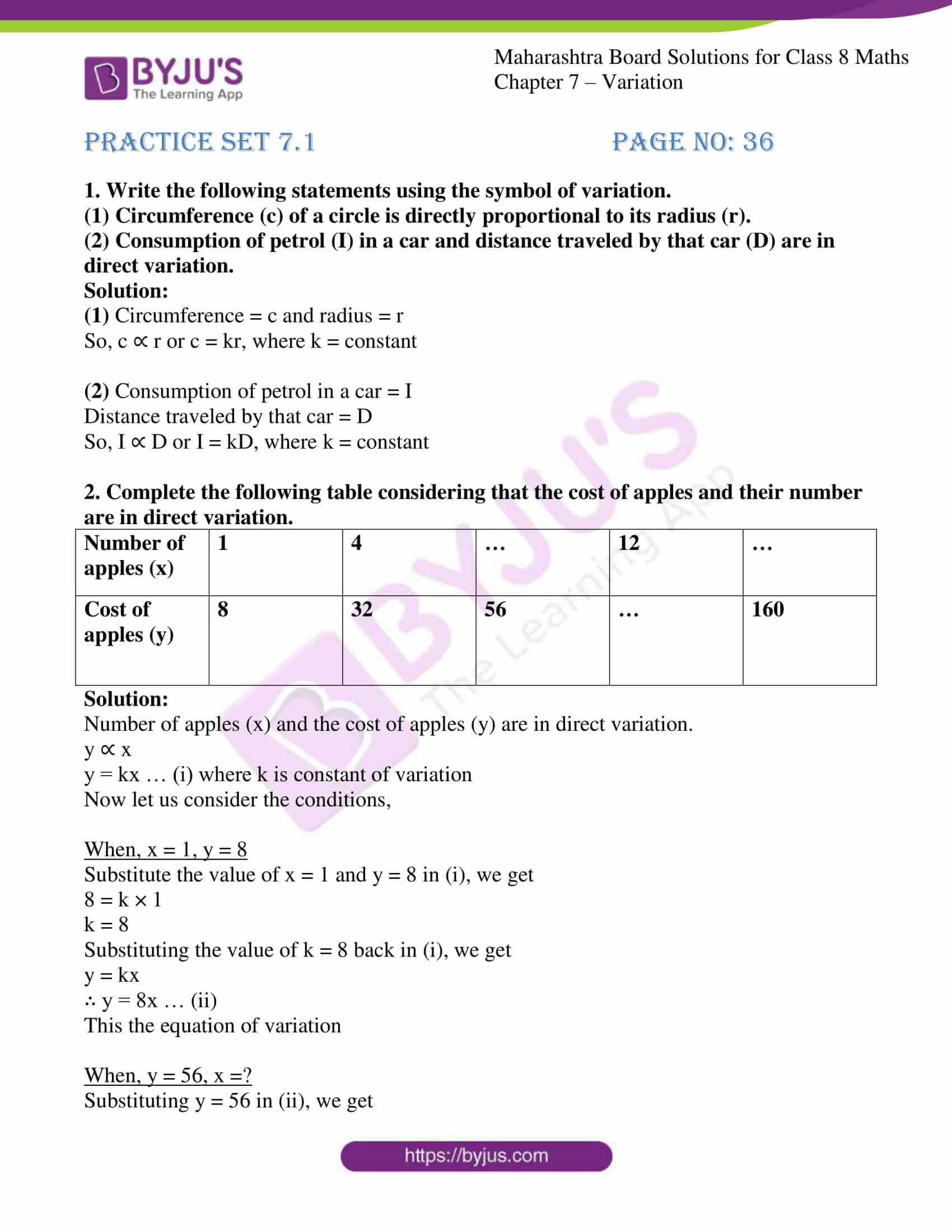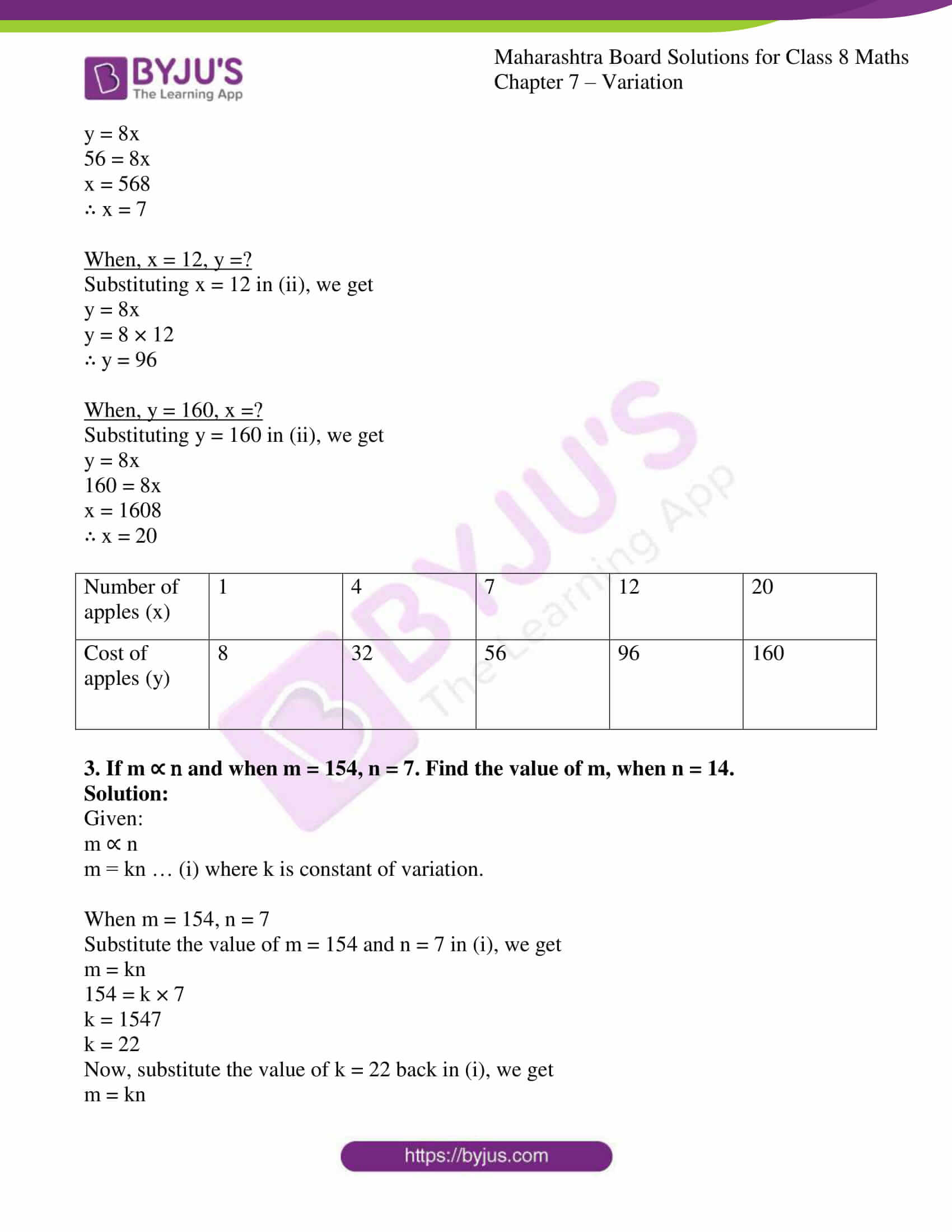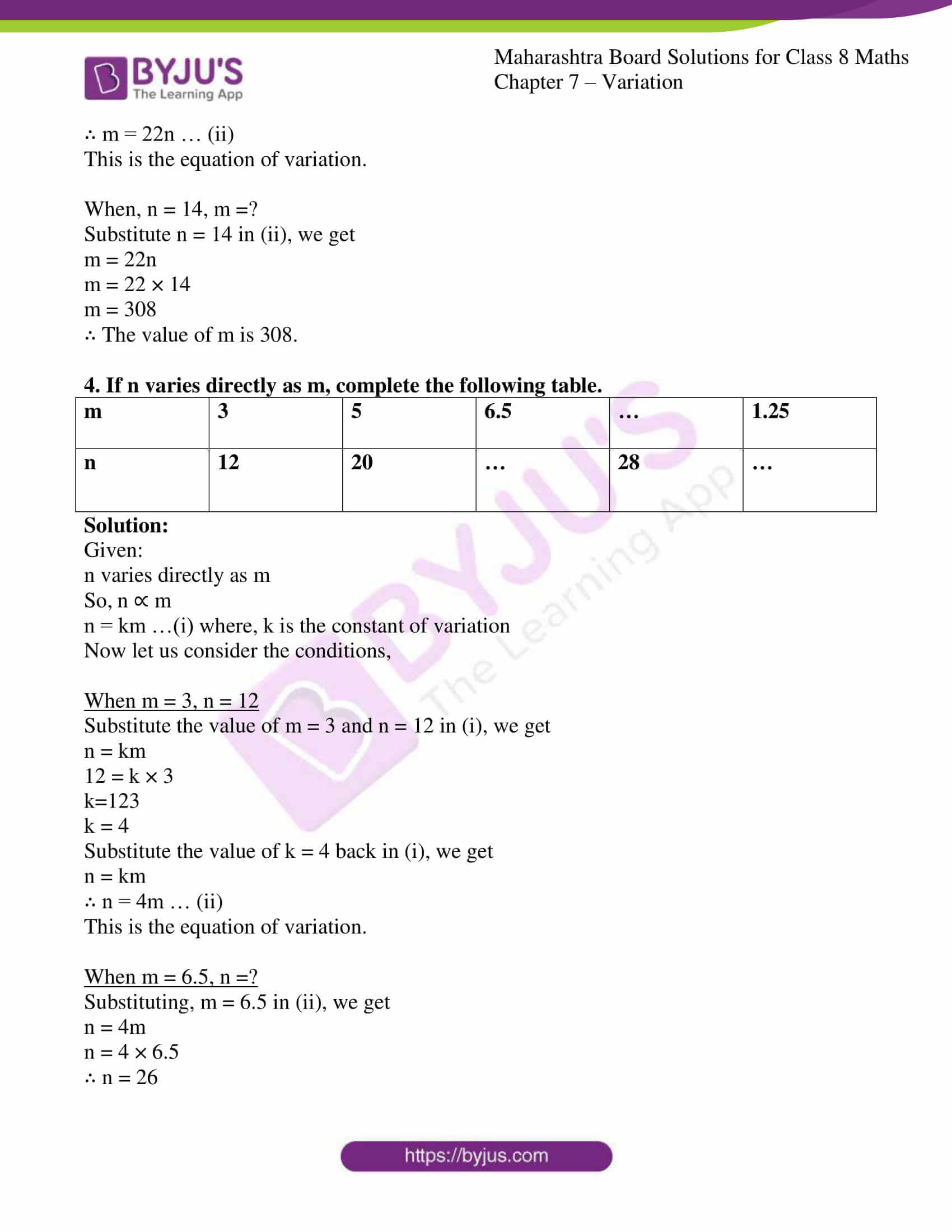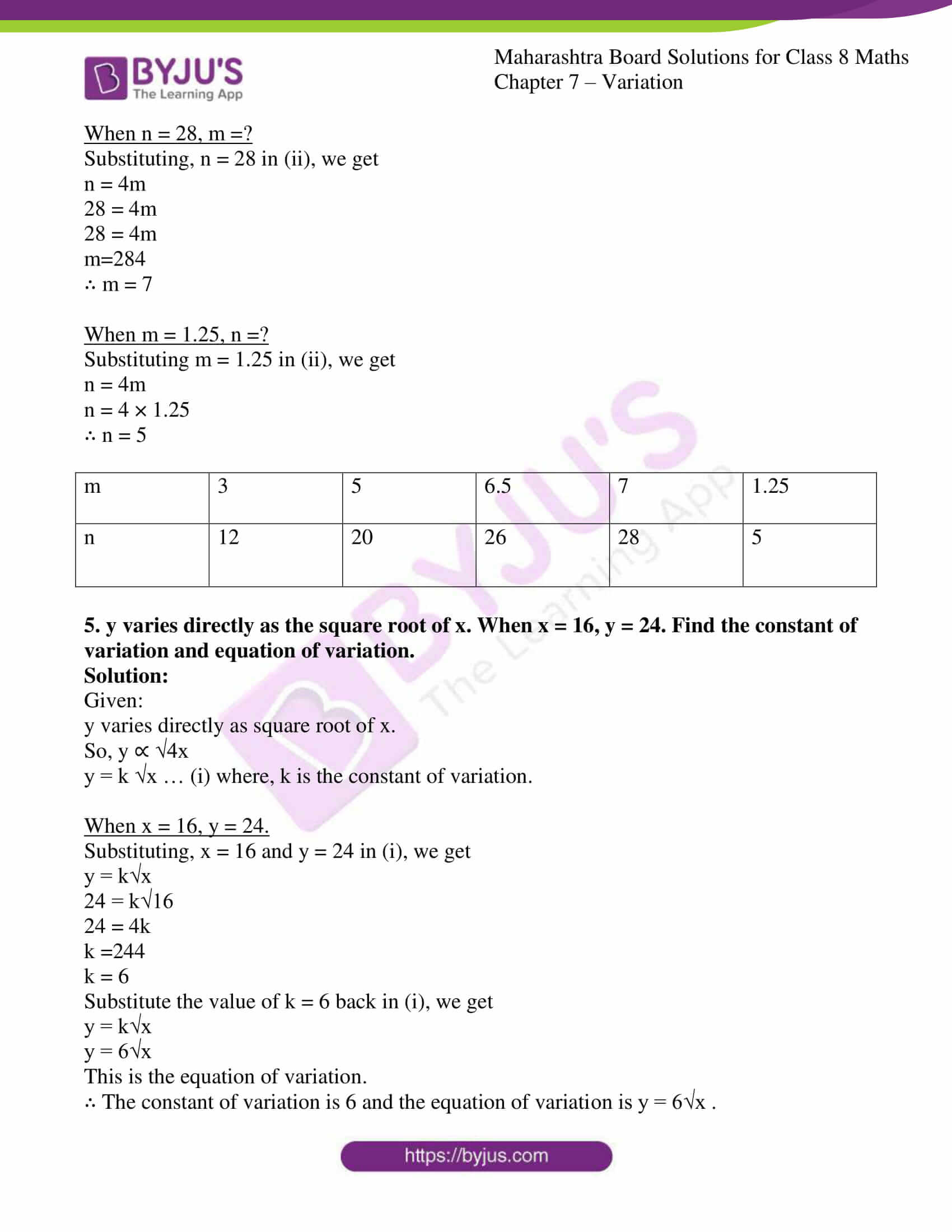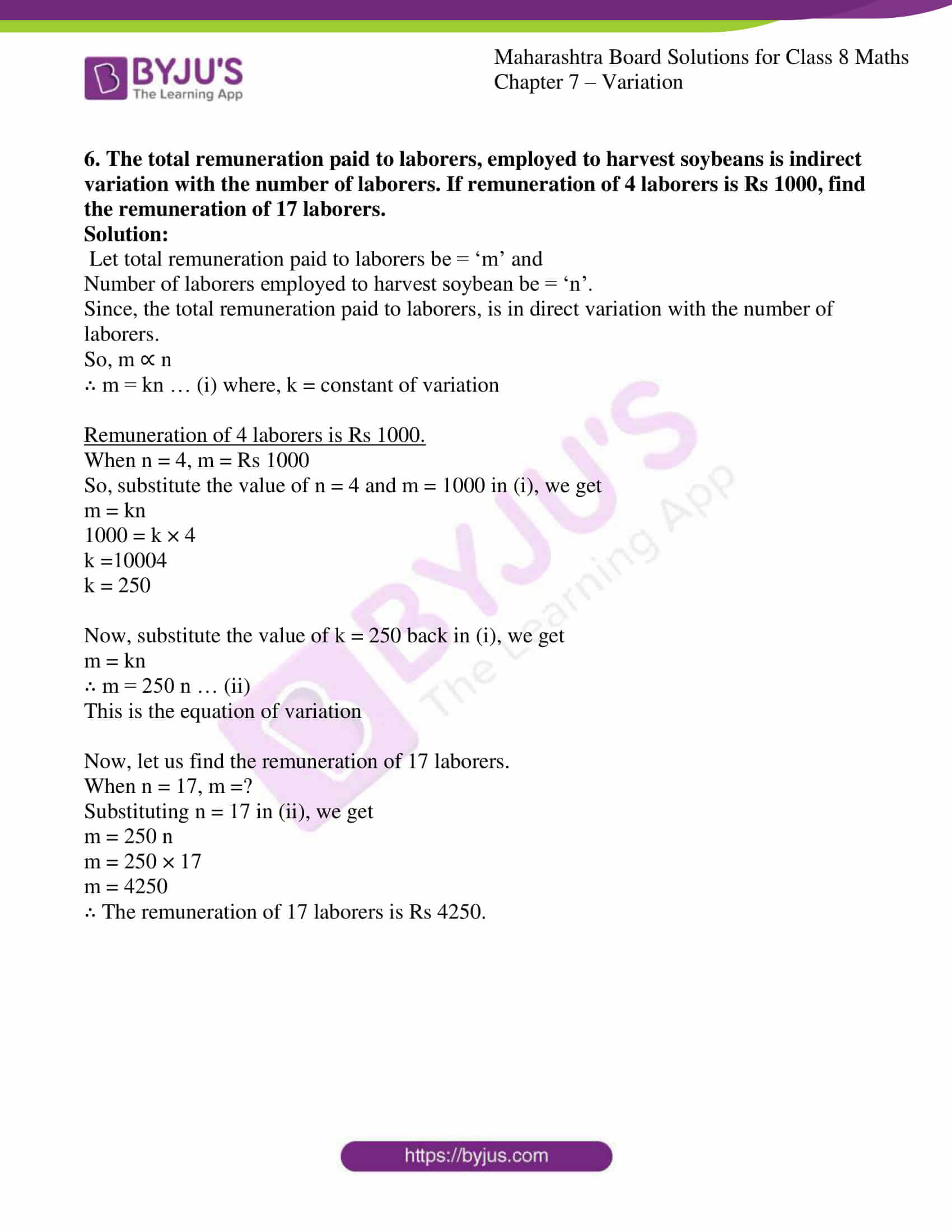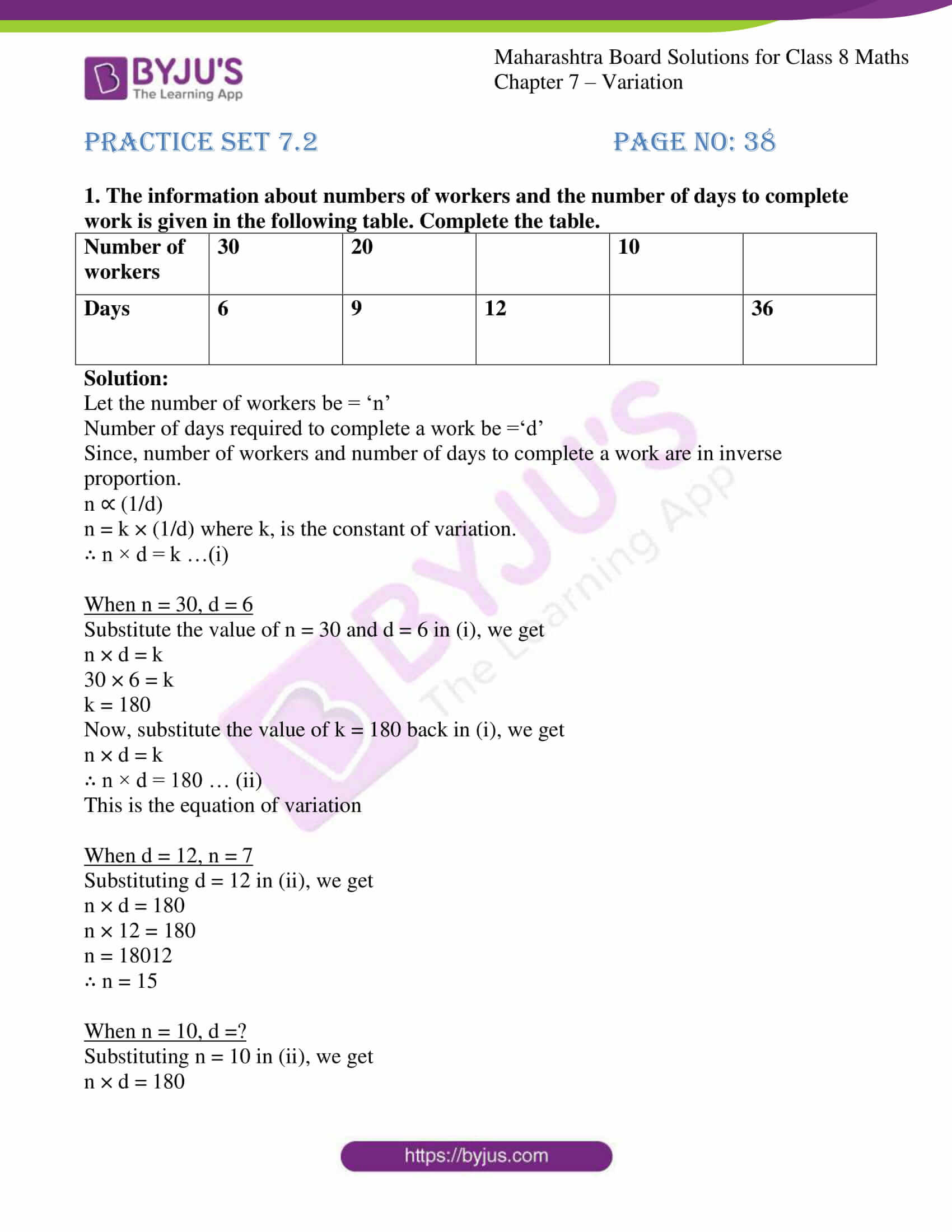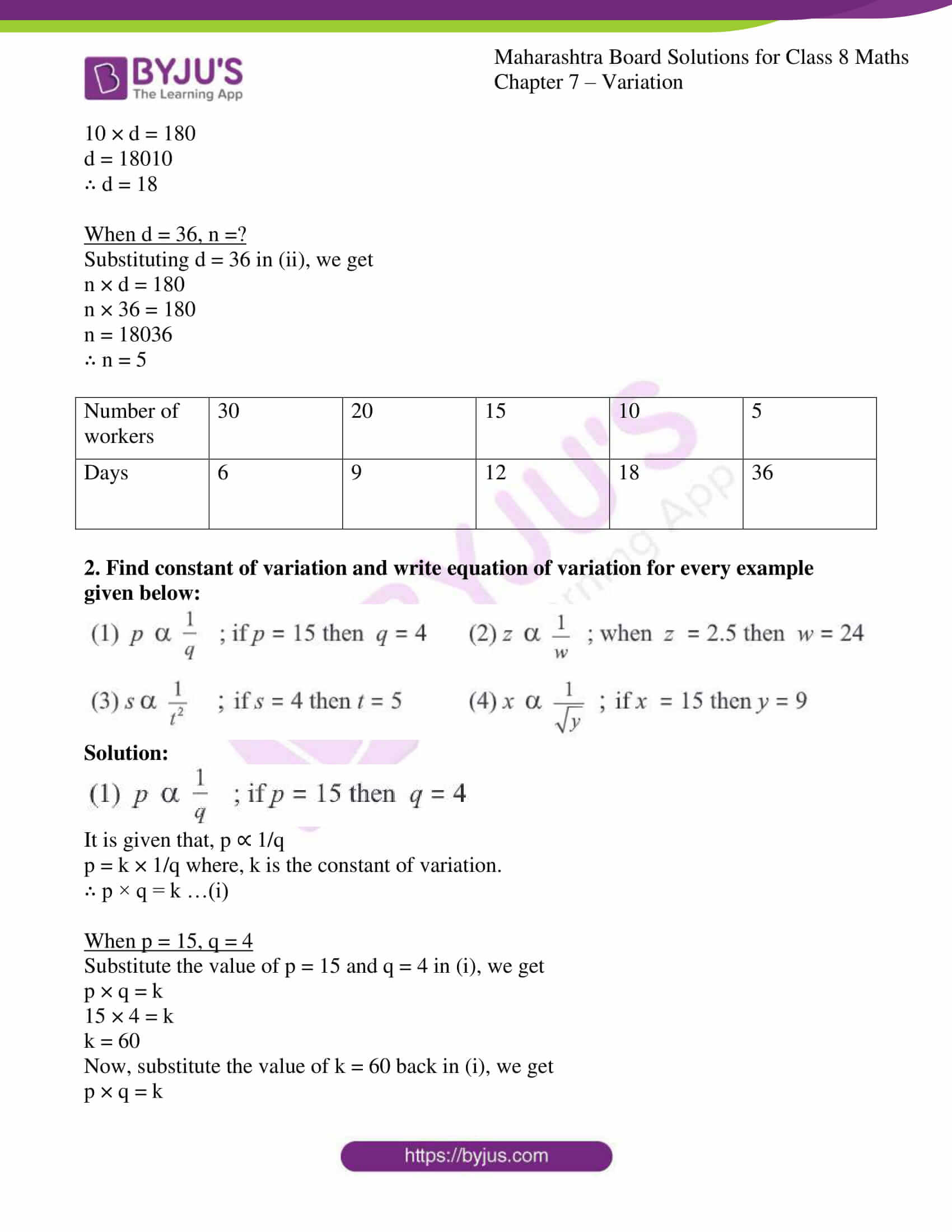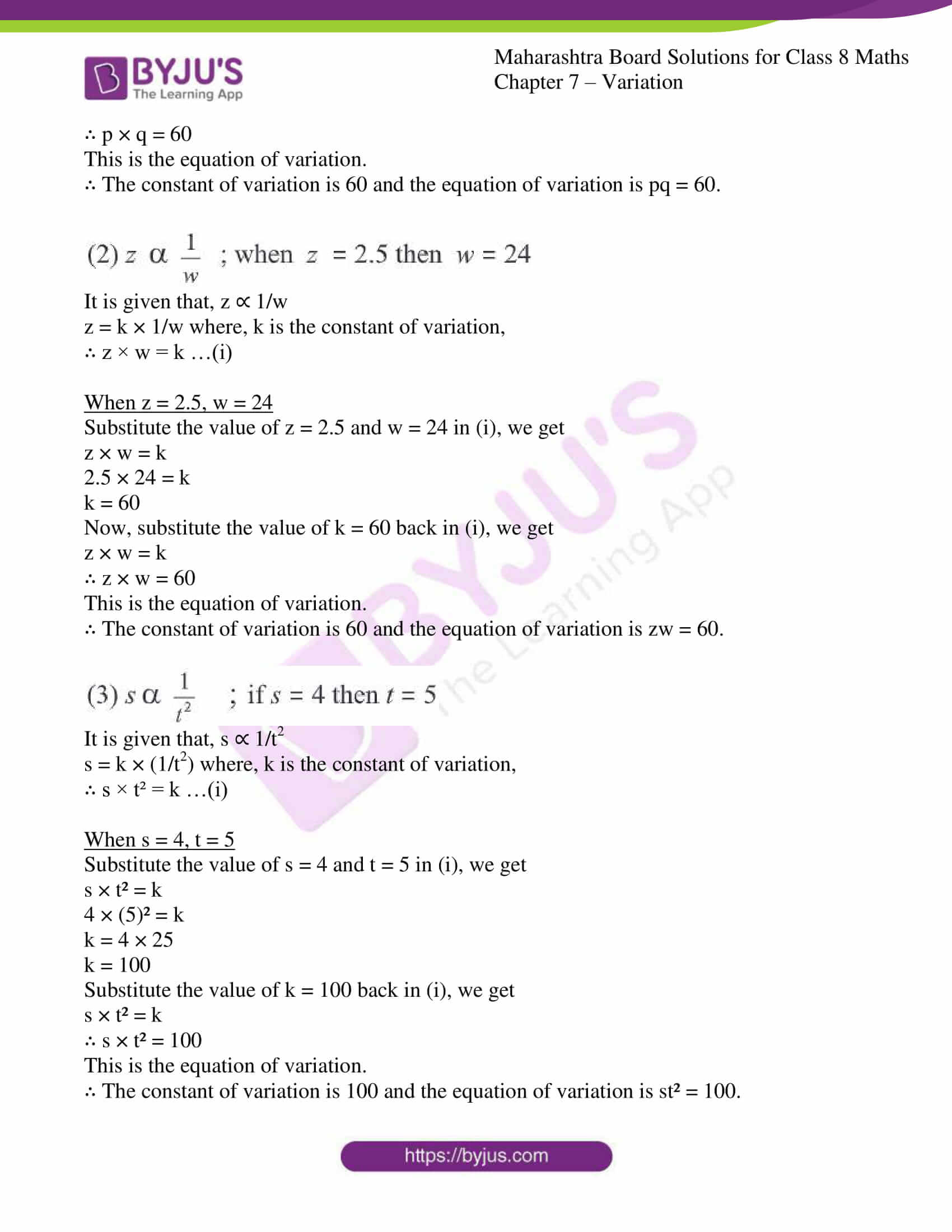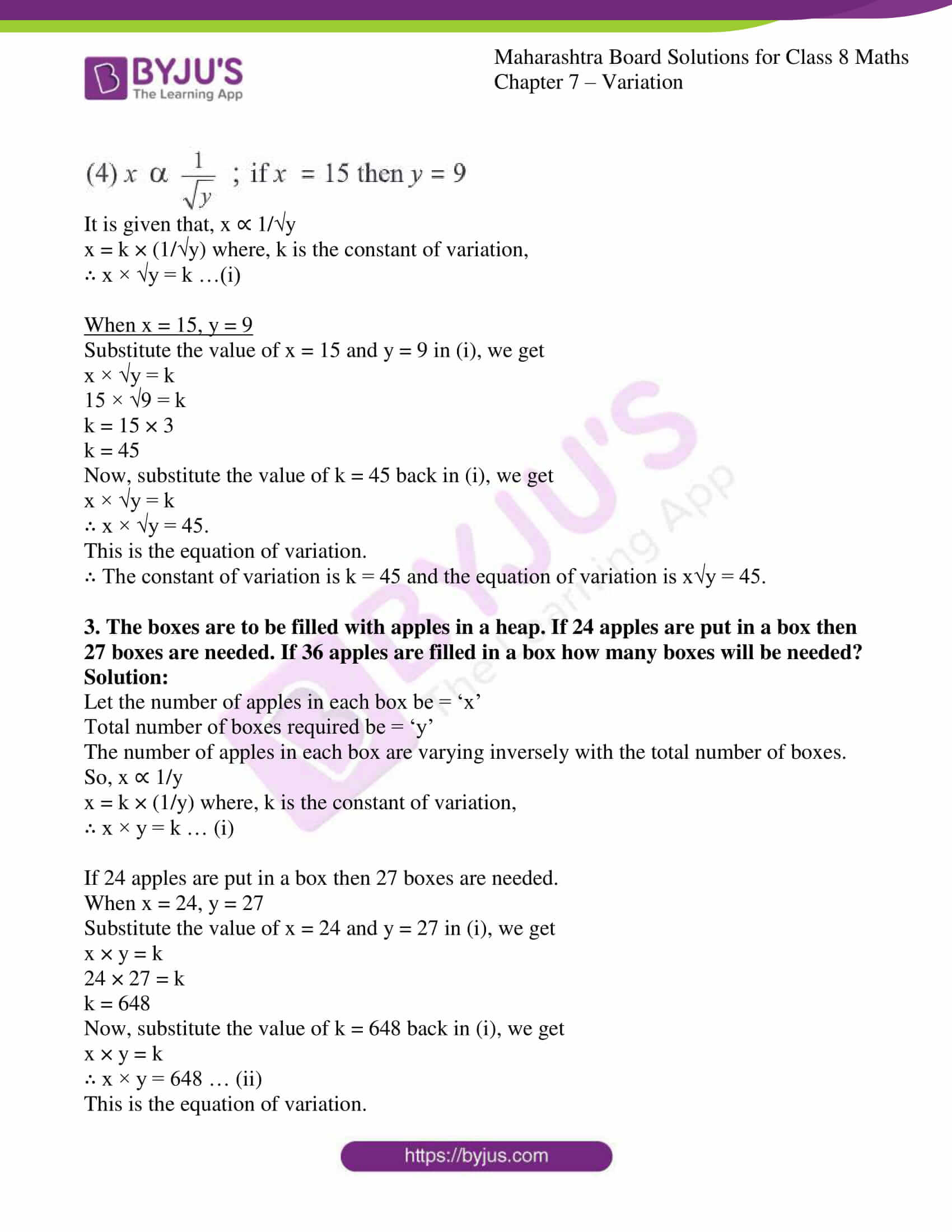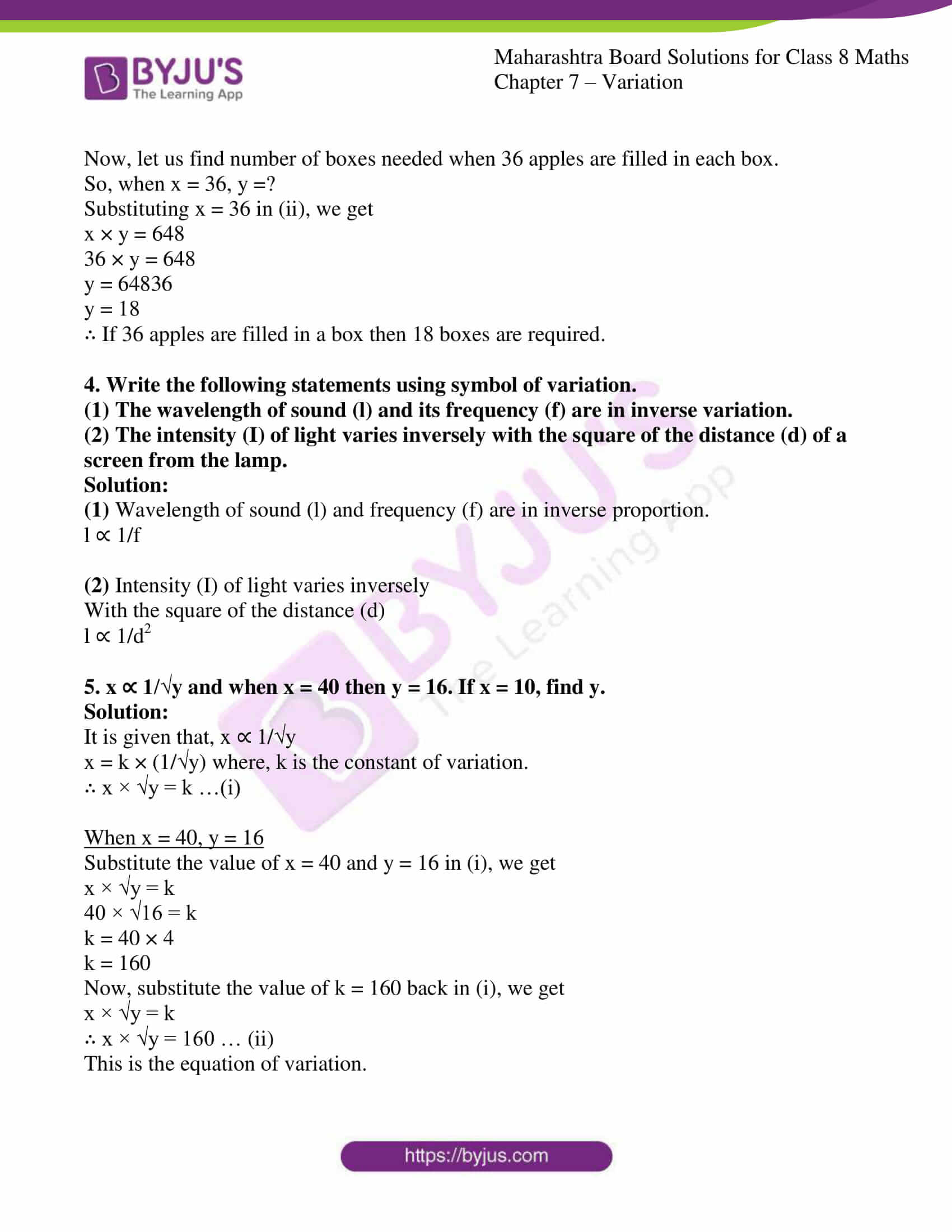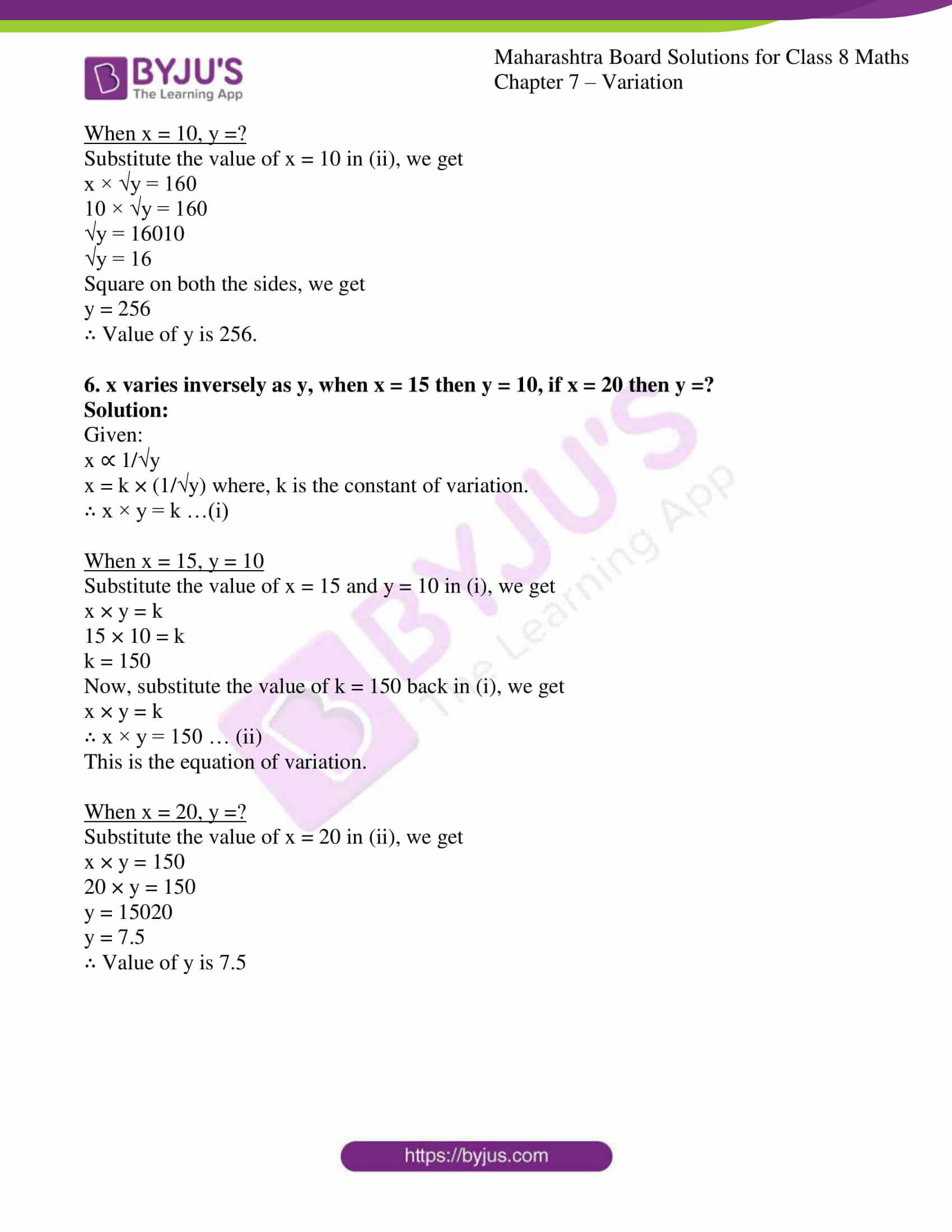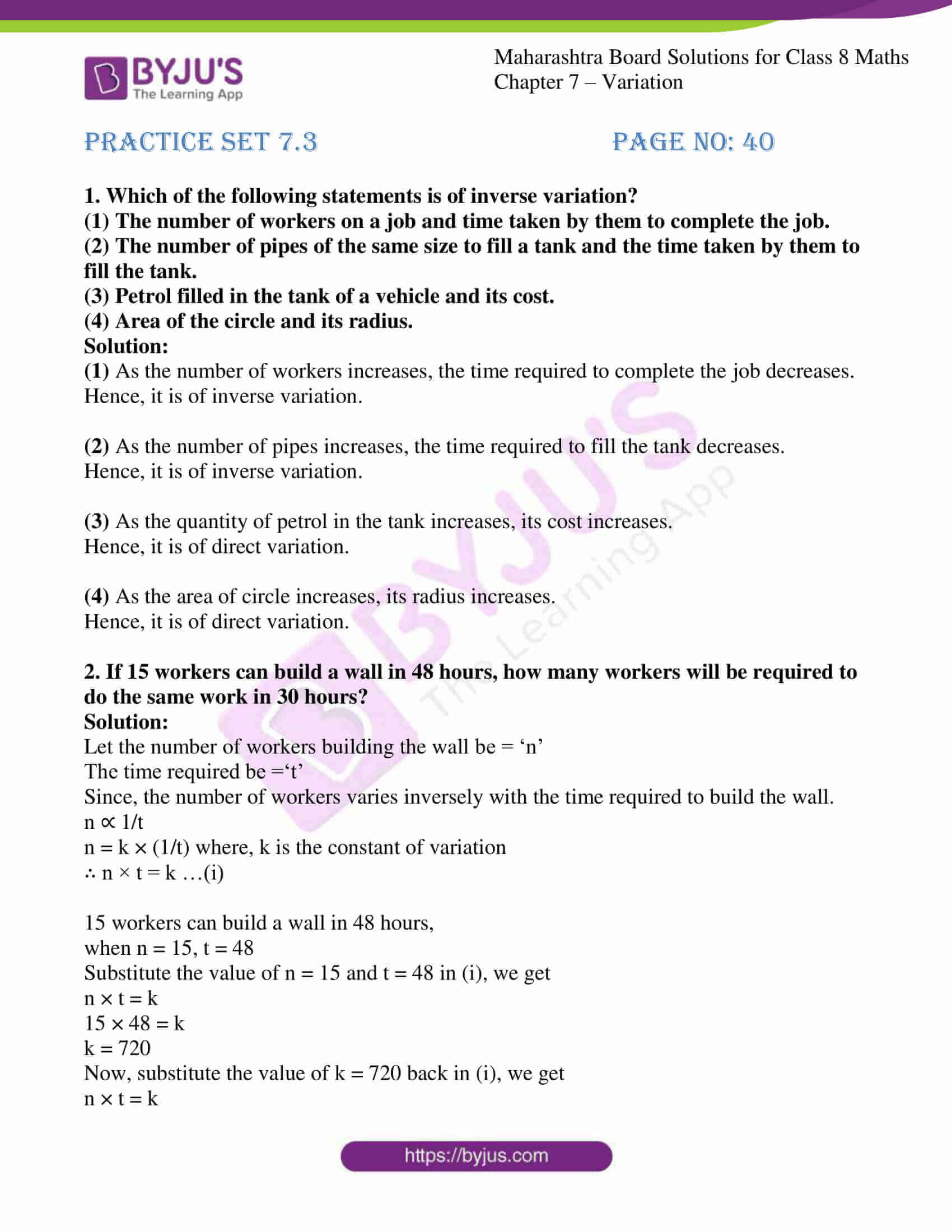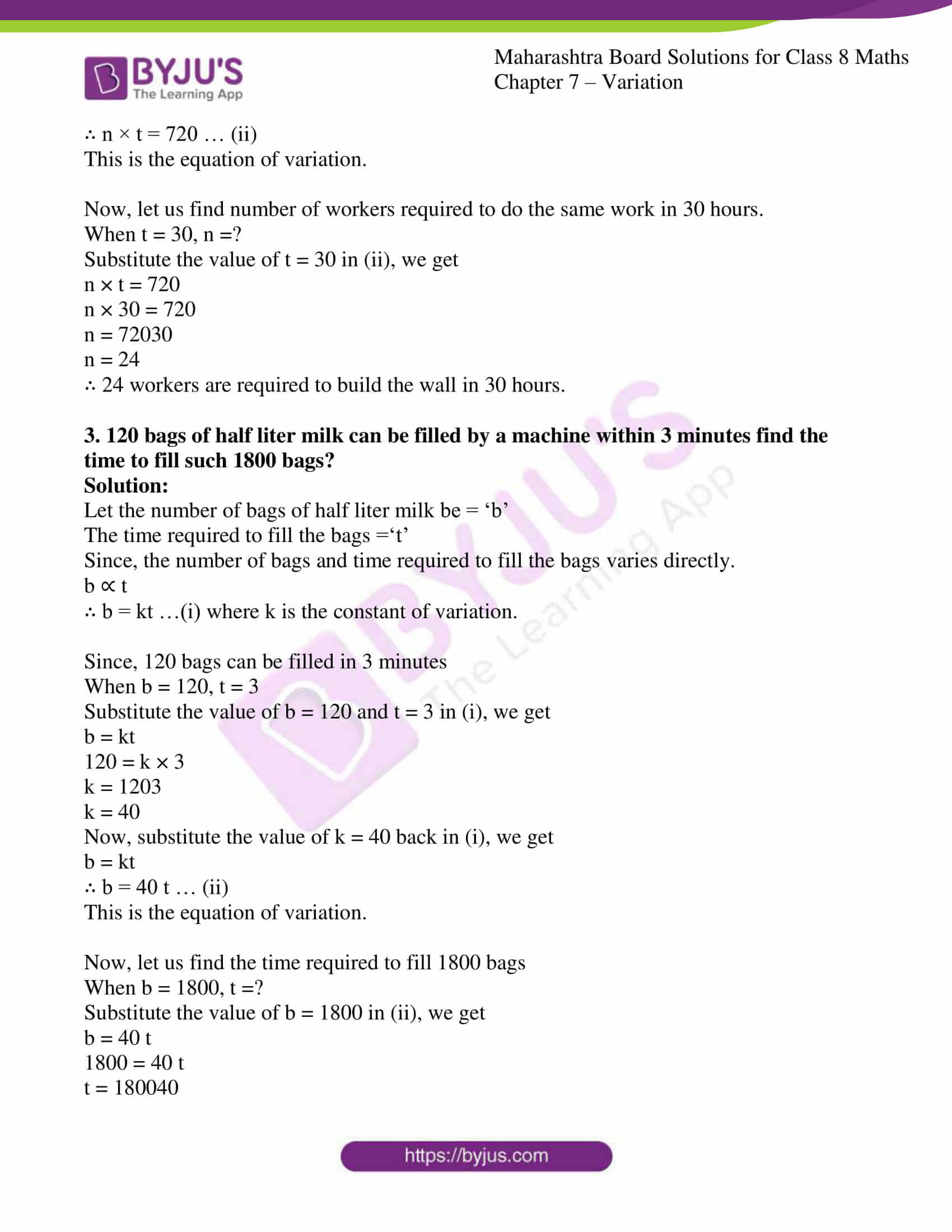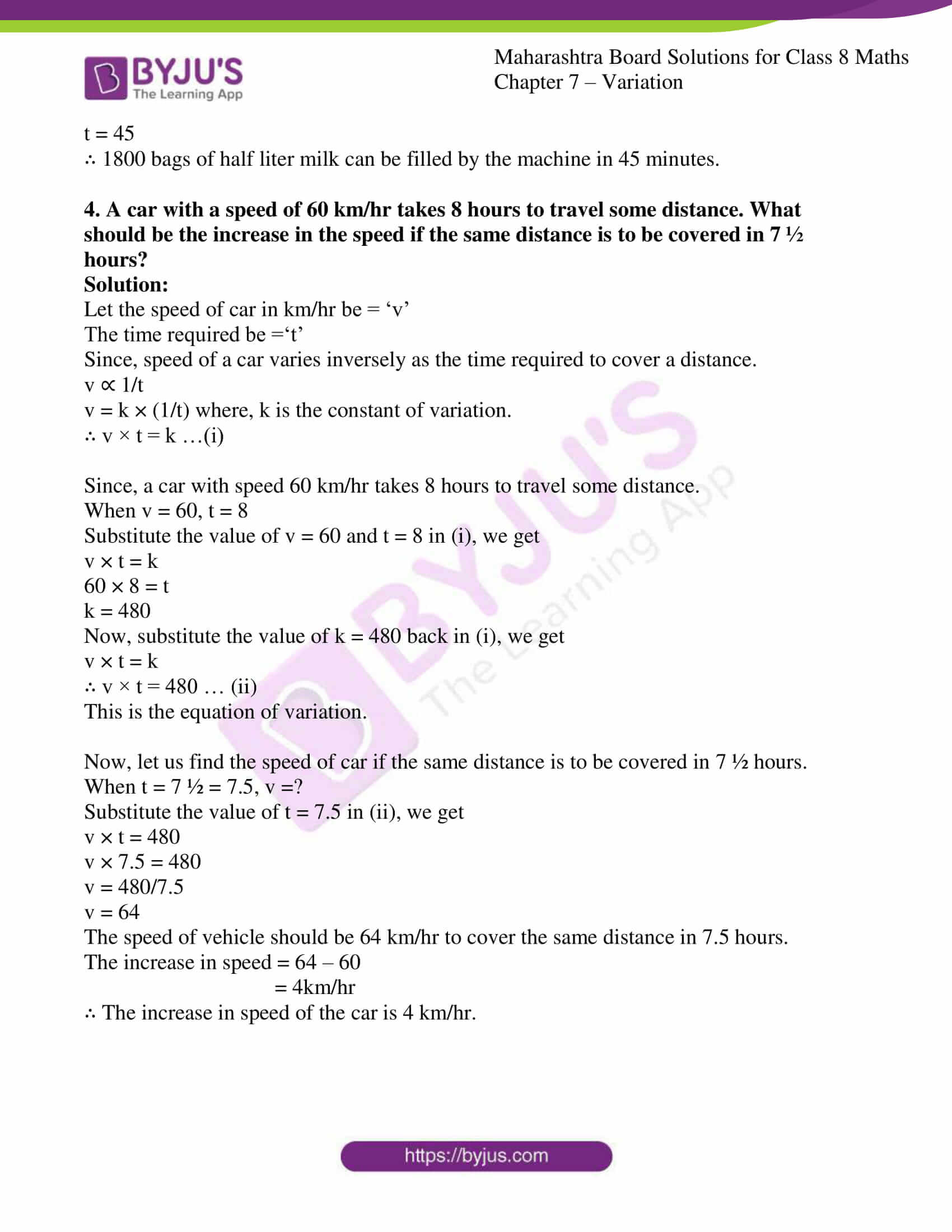### Access answers to Maharashtra Board Solutions For Class 8 Maths Part 1 Chapter 7- Variation.

Practice set 7.1 PAGE NO: 36

1. Write the following statements using the symbol of variation.
(1) Circumference (c) of a circle is directly proportional to its radius (r).
(2) Consumption of petrol (I) in a car and distance traveled by that car (D) are in direct variation.

Solution:

(1) Circumference = c and radius = r

So, c ∝ r or c = kr, where k = constant

(2) Consumption of petrol in a car = I

Distance traveled by that car = D

So, I ∝ D or I = kD, where k = constant

2. Complete the following table considering that the cost of apples and their number are in direct variation.

 Number of apples (x) 1 4 … 12 … Cost of apples (y) 8 32 56 … 160

Solution:

Number of apples (x) and the cost of apples (y) are in direct variation.

y ∝ x

y = kx … (i) where k is constant of variation

Now let us consider the conditions,

When, x = 1, y = 8

Substitute the value of x = 1 and y = 8 in (i), we get

8 = k × 1

k = 8

Substituting the value of k = 8 back in (i), we get

y = kx

∴ y = 8x … (ii)

This the equation of variation

When, y = 56, x =?

Substituting y = 56 in (ii), we get

y = 8x

56 = 8x

x = 568

∴ x = 7

When, x = 12, y =?

Substituting x = 12 in (ii), we get

y = 8x

y = 8 × 12

∴ y = 96

When, y = 160, x =?

Substituting y = 160 in (ii), we get

y = 8x

160 = 8x

x = 1608

∴ x = 20

 Number of apples (x) 1 4 7 12 20 Cost of apples (y) 8 32 56 96 160

3. If m ∝ n and when m = 154, n = 7. Find the value of m, when n = 14.

Solution:

Given:

m ∝ n

m = kn … (i) where k is constant of variation.

When m = 154, n = 7

Substitute the value of m = 154 and n = 7 in (i), we get

m = kn

154 = k × 7

k = 1547

k = 22

Now, substitute the value of k = 22 back in (i), we get

m = kn

∴ m = 22n … (ii)

This is the equation of variation.

When, n = 14, m =?

Substitute n = 14 in (ii), we get

m = 22n

m = 22 × 14

m = 308

∴ The value of m is 308.

4. If n varies directly as m, complete the following table.

 m 3 5 6.5 … 1.25 n 12 20 … 28 …

Solution:

Given:

n varies directly as m

So, n ∝ m

n = km …(i) where, k is the constant of variation

Now let us consider the conditions,

When m = 3, n = 12

Substitute the value of m = 3 and n = 12 in (i), we get

n = km

12 = k × 3

k=123

k = 4

Substitute the value of k = 4 back in (i), we get

n = km

∴ n = 4m … (ii)

This is the equation of variation.

When m = 6.5, n =?

Substituting, m = 6.5 in (ii), we get

n = 4m

n = 4 × 6.5

∴ n = 26

When n = 28, m =?

Substituting, n = 28 in (ii), we get

n = 4m

28 = 4m

28 = 4m

m=284

∴ m = 7

When m = 1.25, n =?

Substituting m = 1.25 in (ii), we get

n = 4m

n = 4 × 1.25

∴ n = 5

 m 3 5 6.5 7 1.25 n 12 20 26 28 5

5. y varies directly as the square root of x. When x = 16, y = 24. Find the constant of variation and equation of variation.

Solution:

Given:

y varies directly as square root of x.

So, y ∝ √4x

y = k √x … (i) where, k is the constant of variation.

When x = 16, y = 24.

Substituting, x = 16 and y = 24 in (i), we get

y = k√x

24 = k√16

24 = 4k

k =244

k = 6

Substitute the value of k = 6 back in (i), we get

y = k√x

y = 6√x

This is the equation of variation.

∴ The constant of variation is 6 and the equation of variation is y = 6√x .

6. The total remuneration paid to laborers, employed to harvest soybeans is indirect variation with the number of laborers. If remuneration of 4 laborers is Rs 1000, find the remuneration of 17 laborers.

Solution:

Let total remuneration paid to laborers be = ‘m’ and

Number of laborers employed to harvest soybean be = ‘n’.

Since, the total remuneration paid to laborers, is in direct variation with the number of laborers.

So, m ∝ n

∴ m = kn … (i) where, k = constant of variation

Remuneration of 4 laborers is Rs 1000.

When n = 4, m = Rs 1000

So, substitute the value of n = 4 and m = 1000 in (i), we get

m = kn

1000 = k × 4

k =10004

k = 250

Now, substitute the value of k = 250 back in (i), we get

m = kn

∴ m = 250 n … (ii)

This is the equation of variation

Now, let us find the remuneration of 17 laborers.

When n = 17, m =?

Substituting n = 17 in (ii), we get

m = 250 n

m = 250 × 17

m = 4250

∴ The remuneration of 17 laborers is Rs 4250.

Practice set 7.2 PAGE NO: 38

1. The information about numbers of workers and the number of days to complete work is given in the following table. Complete the table.

 Number of workers 30 20 10 Days 6 9 12 36

Solution:

Let the number of workers be = ‘n’

Number of days required to complete a work be =‘d’

Since, number of workers and number of days to complete a work are in inverse proportion.

n ∝ (1/d)

n = k × (1/d) where k, is the constant of variation.

∴ n × d = k …(i)

When n = 30, d = 6

Substitute the value of n = 30 and d = 6 in (i), we get

n × d = k

30 × 6 = k

k = 180

Now, substitute the value of k = 180 back in (i), we get

n × d = k

∴ n × d = 180 … (ii)

This is the equation of variation

When d = 12, n = 7

Substituting d = 12 in (ii), we get

n × d = 180

n × 12 = 180

n = 18012

∴ n = 15

When n = 10, d =?

Substituting n = 10 in (ii), we get

n × d = 180

10 × d = 180

d = 18010

∴ d = 18

When d = 36, n =?

Substituting d = 36 in (ii), we get

n × d = 180

n × 36 = 180

n = 18036

∴ n = 5

 Number of workers 30 20 15 10 5 Days 6 9 12 18 36

2. Find constant of variation and write equation of variation for every example given below: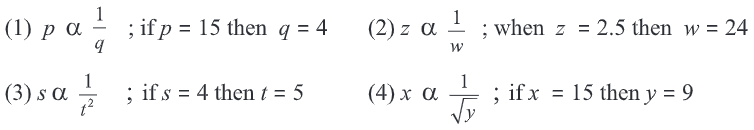Solution: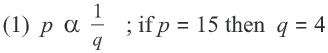It is given that, p ∝ 1/q

p = k × 1/q where, k is the constant of variation.

∴ p × q = k …(i)

When p = 15, q = 4

Substitute the value of p = 15 and q = 4 in (i), we get

p × q = k

15 × 4 = k

k = 60

Now, substitute the value of k = 60 back in (i), we get

p × q = k

∴ p × q = 60

This is the equation of variation.

∴ The constant of variation is 60 and the equation of variation is pq = 60.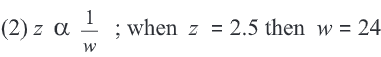It is given that, z ∝ 1/w

z = k × 1/w where, k is the constant of variation,

∴ z × w = k …(i)

When z = 2.5, w = 24

Substitute the value of z = 2.5 and w = 24 in (i), we get

z × w = k

2.5 × 24 = k

k = 60

Now, substitute the value of k = 60 back in (i), we get

z × w = k

∴ z × w = 60

This is the equation of variation.

∴ The constant of variation is 60 and the equation of variation is zw = 60.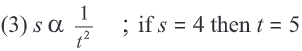It is given that, s ∝ 1/t2

s = k × (1/t2) where, k is the constant of variation,

∴ s × t² = k …(i)

When s = 4, t = 5

Substitute the value of s = 4 and t = 5 in (i), we get

s × t² = k

4 × (5)² = k

k = 4 × 25

k = 100

Substitute the value of k = 100 back in (i), we get

s × t² = k

∴ s × t² = 100

This is the equation of variation.

∴ The constant of variation is 100 and the equation of variation is st² = 100.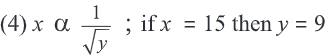It is given that, x ∝ 1/√y

x = k × (1/√y) where, k is the constant of variation,

∴ x × √y = k …(i)

When x = 15, y = 9

Substitute the value of x = 15 and y = 9 in (i), we get

x × √y = k

15 × √9 = k

k = 15 × 3

k = 45

Now, substitute the value of k = 45 back in (i), we get

x × √y = k

∴ x × √y = 45.

This is the equation of variation.

∴ The constant of variation is k = 45 and the equation of variation is x√y = 45.

3. The boxes are to be filled with apples in a heap. If 24 apples are put in a box then 27 boxes are needed. If 36 apples are filled in a box how many boxes will be needed?

Solution:

Let the number of apples in each box be = ‘x’

Total number of boxes required be = ‘y’

The number of apples in each box are varying inversely with the total number of boxes.

So, x ∝ 1/y

x = k × (1/y) where, k is the constant of variation,

∴ x × y = k … (i)

If 24 apples are put in a box then 27 boxes are needed.

When x = 24, y = 27

Substitute the value of x = 24 and y = 27 in (i), we get

x × y = k

24 × 27 = k

k = 648

Now, substitute the value of k = 648 back in (i), we get

x × y = k

∴ x × y = 648 … (ii)

This is the equation of variation.

Now, let us find number of boxes needed when 36 apples are filled in each box.

So, when x = 36, y =?

Substituting x = 36 in (ii), we get

x × y = 648

36 × y = 648

y = 64836

y = 18

∴ If 36 apples are filled in a box then 18 boxes are required.

4. Write the following statements using symbol of variation.

(1) The wavelength of sound (l) and its frequency (f) are in inverse variation.

(2) The intensity (I) of light varies inversely with the square of the distance (d) of a screen from the lamp.

Solution:

(1) Wavelength of sound (l) and frequency (f) are in inverse proportion.

l ∝ 1/f

(2) Intensity (I) of light varies inversely

With the square of the distance (d)

l ∝ 1/d2

5. x ∝ 1/√y and when x = 40 then y = 16. If x = 10, find y.

Solution:

It is given that, x ∝ 1/√y

x = k × (1/√y) where, k is the constant of variation.

∴ x × √y = k …(i)

When x = 40, y = 16

Substitute the value of x = 40 and y = 16 in (i), we get

x × √y = k

40 × √16 = k

k = 40 × 4

k = 160

Now, substitute the value of k = 160 back in (i), we get

x × √y = k

∴ x × √y = 160 … (ii)

This is the equation of variation.

When x = 10, y =?

Substitute the value of x = 10 in (ii), we get

x × √y = 160

10 × √y = 160

√y = 16010

√y = 16

Square on both the sides, we get

y = 256

∴ Value of y is 256.

6. x varies inversely as y, when x = 15 then y = 10, if x = 20 then y =?

Solution:

Given:

x ∝ 1/√y

x = k × (1/√y) where, k is the constant of variation.

∴ x × y = k …(i)

When x = 15, y = 10

Substitute the value of x = 15 and y = 10 in (i), we get

x × y = k

15 × 10 = k

k = 150

Now, substitute the value of k = 150 back in (i), we get

x × y = k

∴ x × y = 150 … (ii)

This is the equation of variation.

When x = 20, y =?

Substitute the value of x = 20 in (ii), we get

x × y = 150

20 × y = 150

y = 15020

y = 7.5

∴ Value of y is 7.5

Practice set 7.3 PAGE NO: 40

1. Which of the following statements is of inverse variation?
(1) The number of workers on a job and time taken by them to complete the job.
(2) The number of pipes of the same size to fill a tank and the time taken by them to fill the tank.
(3) Petrol filled in the tank of a vehicle and its cost.
(4) Area of the circle and its radius.

Solution:

(1) As the number of workers increases, the time required to complete the job decreases.

Hence, it is of inverse variation.

(2) As the number of pipes increases, the time required to fill the tank decreases.

Hence, it is of inverse variation.

(3) As the quantity of petrol in the tank increases, its cost increases.

Hence, it is of direct variation.

(4) As the area of circle increases, its radius increases.

Hence, it is of direct variation.

2. If 15 workers can build a wall in 48 hours, how many workers will be required to do the same work in 30 hours?

Solution:

Let the number of workers building the wall be = ‘n’

The time required be =‘t’

Since, the number of workers varies inversely with the time required to build the wall.

n ∝ 1/t

n = k × (1/t) where, k is the constant of variation

∴ n × t = k …(i)

15 workers can build a wall in 48 hours,

when n = 15, t = 48

Substitute the value of n = 15 and t = 48 in (i), we get

n × t = k

15 × 48 = k

k = 720

Now, substitute the value of k = 720 back in (i), we get

n × t = k

∴ n × t = 720 … (ii)

This is the equation of variation.

Now, let us find number of workers required to do the same work in 30 hours.

When t = 30, n =?

Substitute the value of t = 30 in (ii), we get

n × t = 720

n × 30 = 720

n = 72030

n = 24

∴ 24 workers are required to build the wall in 30 hours.

3. 120 bags of half liter milk can be filled by a machine within 3 minutes find the time to fill such 1800 bags?

Solution:

Let the number of bags of half liter milk be = ‘b’

The time required to fill the bags =‘t’

Since, the number of bags and time required to fill the bags varies directly.

b ∝ t

∴ b = kt …(i) where k is the constant of variation.

Since, 120 bags can be filled in 3 minutes

When b = 120, t = 3

Substitute the value of b = 120 and t = 3 in (i), we get

b = kt

120 = k × 3

k = 1203

k = 40

Now, substitute the value of k = 40 back in (i), we get

b = kt

∴ b = 40 t … (ii)

This is the equation of variation.

Now, let us find the time required to fill 1800 bags

When b = 1800, t =?

Substitute the value of b = 1800 in (ii), we get

b = 40 t

1800 = 40 t

t = 180040

t = 45

∴ 1800 bags of half liter milk can be filled by the machine in 45 minutes.

4. A car with a speed of 60 km/hr takes 8 hours to travel some distance. What should be the increase in the speed if the same distance is to be covered in 7 ½ hours?

Solution:

Let the speed of car in km/hr be = ‘v’

The time required be =‘t’

Since, speed of a car varies inversely as the time required to cover a distance.

v ∝ 1/t

v = k × (1/t) where, k is the constant of variation.

∴ v × t = k …(i)

Since, a car with speed 60 km/hr takes 8 hours to travel some distance.

When v = 60, t = 8

Substitute the value of v = 60 and t = 8 in (i), we get

v × t = k

60 × 8 = t

k = 480

Now, substitute the value of k = 480 back in (i), we get

v × t = k

∴ v × t = 480 … (ii)

This is the equation of variation.

Now, let us find the speed of car if the same distance is to be covered in 7 ½ hours.

When t = 7 ½ = 7.5, v =?

Substitute the value of t = 7.5 in (ii), we get

v × t = 480

v × 7.5 = 480

v = 480/7.5

v = 64

The speed of vehicle should be 64 km/hr to cover the same distance in 7.5 hours.

The increase in speed = 64 – 60

= 4km/hr

∴ The increase in speed of the car is 4 km/hr.

Students can refer to MSBSHSE Solutions to have a good hold of the concepts covered in this chapter. Chapter 7 Variation, which includes Direct variation and Inverse variations provides useful resources for students as it helps them in scoring full marks in the examination. Many such exercise problems are given for students to practice and to secure good marks in their examination.

## Frequently Asked Questions on Maharashtra State Board Solutions for Class 8 Maths Chapter 7 Variation

### Are these Maharashtra Board Class 8 Maths Chapter 7 Variation Solutions useful to prepare for the exams?

Yes, these Maharashtra Board Class 8 Maths Chapter 7 Variation Solutions can be very helpful. Students are highly recommended to practise these solutions after revising the subjects, as they set the basis for the questions that could get asked often in the board exams.

### How are these Maharashtra State Board Class 8 Maths Chapter 7 Variation Solutions helpful?

Students are encouraged to download these solutions and practise them. Then they can refer back to the solutions to analyse their performance. This will also help them to rectify their mistakes and to avoid making them during the board exams.

### How can we find these Maharashtra Board Class 8 Maths Chapter 7 Solutions?

We provide the solutions to download. We have also given the solutions as a scrollable PDF and we have also mentioned the clickable link for the students to access. Meanwhile, the questions and the solutions  are also available online on our webpage.